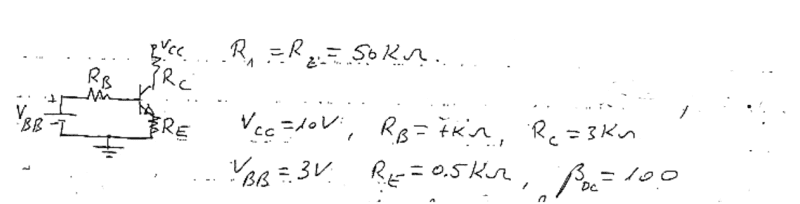# Transistor circut analysis

Hi there! I'm having trouble understanding the transistor circuit analysis. Hope you could help me :)First I need to find the active region of the transistor. What I saw in the solutions was an assumption that the transistor is in saturation region and then:

VBB=IbRb+Vbe+IeRe
Vcc=IcRc+Vce+IeRe

Why did the solver assume the transistor is in saturation mode, when he is supposed to find the active region?

Next, I have to calculate the minimal RE needed for the transistor to be active.
This time, they did refer to it as in active region. But, the equations remained the same. I thought that in Active region, it is supposed to reverse:

VBB=IbRb+Vbe+IeRe
Vce-IcRc-Vcc=IeRe

Why isn't there any change in the equations ? the BC diode is supposed to be in reverse mode, isn't it ?

Thank you!

NascentOxygen
Staff Emeritus
First I need to find the active region of the transistor. What I saw in the solutions was an assumption that the transistor is in saturation region and then:

VBB=IbRb+Vbe+IeRe
Vcc=IcRc+Vce+IeRe

Why did the solver assume the transistor is in saturation mode, when he is supposed to find the active region?
There is nothing here to indicate an assumption of saturation. Those equations hold for both regions of operation, active and saturated.
Why isn't there any change in the equations ? the BC diode is supposed to be in reverse mode, isn't it ?
Yes, and that condition is implicit in setting VCE = 0. (Explanation: If VCE = 0 and VBE = 0.6, then it follows that VCE = -0.6)

[PLAIN]https://www.physicsforums.com/images/icons/icon2.gifAlso, [Broken] may I point out that you should not be using lower-case subscripts here, as they mean something different from the capitals subscripts. For DC or average values, use upper-case subscripts.

Last edited by a moderator:
rude man
Homework Helper
Gold Member
Hi there! I'm having trouble understanding the transistor circuit analysis. Hope you could help me :)
View attachment 50224

First I need to find the active region of the transistor. What I saw in the solutions was an assumption that the transistor is in saturation region and then:

VBB=IbRb+Vbe+IeRe
Vcc=IcRc+Vce+IeRe

Why did the solver assume the transistor is in saturation mode, when he is supposed to find the active region?

Next, I have to calculate the minimal RE needed for the transistor to be active.
This time, they did refer to it as in active region. But, the equations remained the same. I thought that in Active region, it is supposed to reverse:

VBB=IbRb+Vbe+IeRe
Vce-IcRc-Vcc=IeRe

Why isn't there any change in the equations ? the BC diode is supposed to be in reverse mode, isn't it ?

Thank you!

The first set of 2 equations is correct irrespective of values of the R's or power supply voltages. The second set is garbage.

A quick check shows that this configuration IS in saturation. Approximately,
ie = (3V-0.7V)/0.5K = 4.6 mA which when multiplied by Rc = 3K gives Vcc - Vc = 13.8V, exceeding even the Vcc power supply.

(How did I do that? I just assumed β = ∞, a reasonable approx. with the low value of Rb).